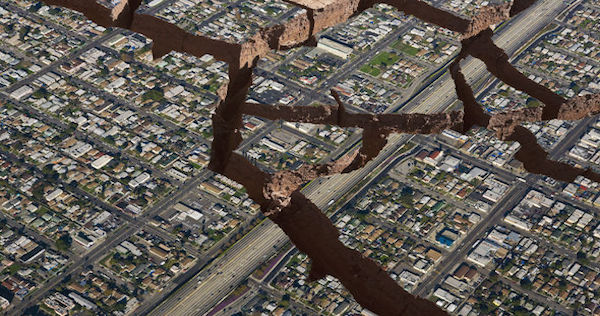# The Richter Scale for measuring earthquake magnitudeThe Richter Scale is a logarithmic scale that has been used for measuring the seismic energy given off by earthquakes since the 1930s. While it serves a scientific purpose when measuring small tremors that occur every day, it’s exponential nature makes it very impractical for use when describing earthquake magnitude in easily quantifiable terms.

We think of a scale as a linear continuum where each segment is equal. Not so on the Richter Scale. On the Richter Scale each whole number increase represents a ten-fold increase in seismic energy. So, a 2 is ten times greater than a 1, and a 7 is 100 times greater than a 5. As you can see, it is difficult to get an accurate sense of magnitude when using the Richter Scale without converting to “real numbers.”

As shown in the chart below, the Japanese earthquake of 2011 scored a record-breaking 9 on the Richter Scale. That seems like a little more that double a 4, when in fact a 4 scores a 1,000 (one thousand) and a 9 scores a 100,000,000 (one hundred million). Quite a change in perspective, wouldn’t you say?

Richter Scale = conversion to “real numbers”

1 = 1

2 = 10

3 = 100

4 = 1,000

5 = 10,000

6 = 100,000

7 = 1,000,000

8 = 10,000,000

9 = 100,000,000

10 = 1,000,000,000.

source: andantepublishing.org

Published in